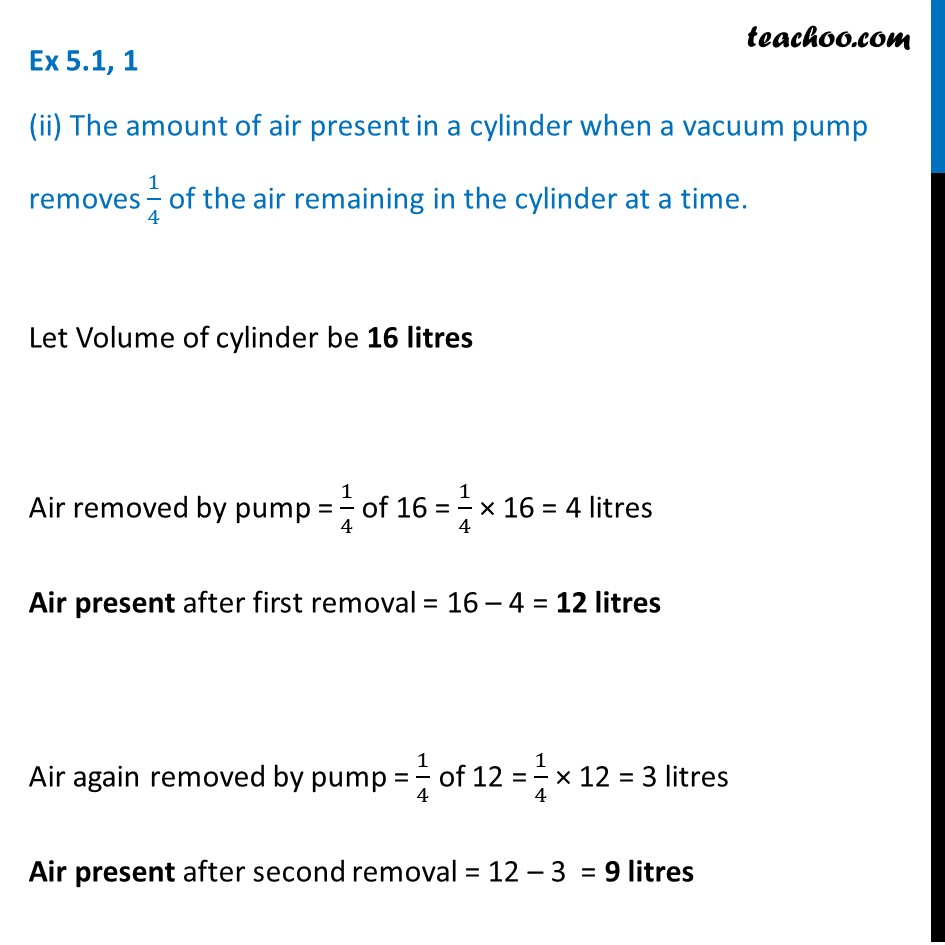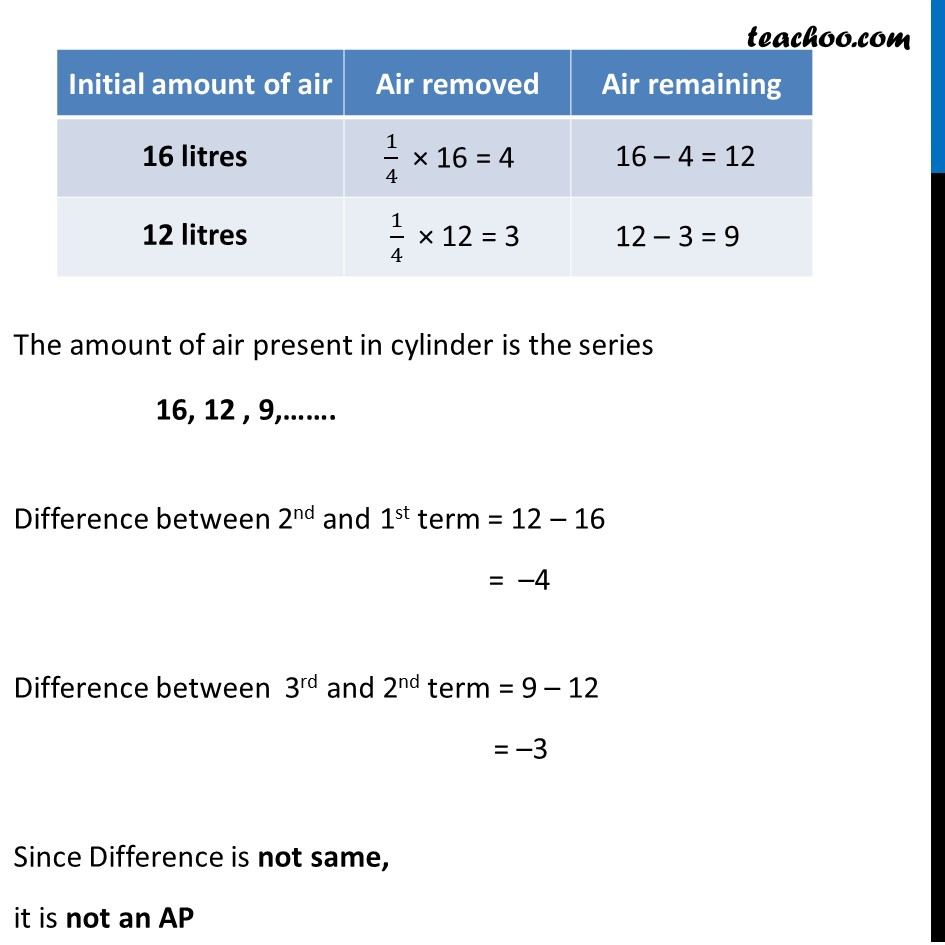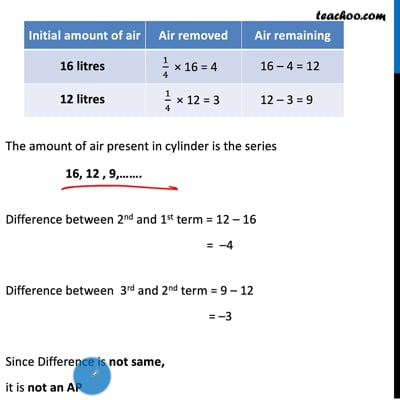Ex 5.1

Chapter 5 Class 10 Arithmetic Progressions
Serial order wiseThis video is only available for Teachoo black users

Introducing your new favourite teacher - Teachoo Black, at only ₹83 per month

### Transcript

Ex 5.1, 1 (ii) The amount of air present in a cylinder when a vacuum pump removes 1/4 of the air remaining in the cylinder at a time. Let Volume of cylinder be 16 litres Air removed by pump = 1/4 of 16 = 1/4 × 16 = 4 litres Air present after first removal = 16 – 4 = 12 litres Air again removed by pump = 1/4 of 12 = 1/4 × 12 = 3 litres Air present after second removal = 12 – 3 = 9 litres The amount of air present in cylinder is the series 16, 12 , 9,……. Difference between 2nd and 1st term = 12 – 16 = –4 Difference between 3rd and 2nd term = 9 – 12 = –3 Since Difference is not same, it is not an AP